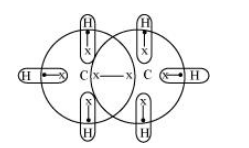Deepak Scored 45->99%ile with Bounce Back Crack Course. You can do it too!

# Do you expect the carbon hydrides of the type

Question:

Do you expect the carbon hydrides of the type $\left(\mathrm{C}_{n} \mathrm{H}_{2 n+2}\right)$ to act as 'Lewis' acid or base? Justify your answer.

Solution:

For carbon hydrides of type $\mathrm{C}_{n} \mathrm{H}_{2 n+2}$, the following hydrides are possible for

$n=1 \Rightarrow \mathrm{CH}_{4}$

$n=2 \Rightarrow \mathrm{C}_{2} \mathrm{H}_{6}$

$n=3 \Rightarrow \mathrm{C}_{3} \mathrm{H}_{8}$

For a hydride to act as a Lewis acid i.e., electron accepting, it should be electron-deficient. Also, for it to act as a Lewis base i.e., electron donating, it should be electron-rich.

Taking $\mathrm{C}_{2} \mathrm{H}_{6}$ as an example, the total number of electrons are 14 and the total covalent bonds are seven. Hence, the bonds are regular $2 \mathrm{e}^{-}-2$ centered bonds.Hence, hydride $\mathrm{C}_{2} \mathrm{H}_{6}$ has sufficient electrons to be represented by a conventional Lewis structure. Therefore, it is an electron-precise hydride, having all atoms with complete octets. Thus, it can neither donate nor accept electrons to act as a Lewis acid or Lewis base.